id:Test#1 MC portion 31 questions- 2points each. Total 60 points (yes 3 extra bonus points). Take your time. Only first submission will be graded.

Reference classes here

 MULTIPLE CHOICE. Choose the one alternative that best completes the statement or answers the question. 1) A Java program is best classified as 1) a b c d e
 A)  input B)  storage C)  hardware D)  software E)  processor

 2) 6 bits can be used to represent ________ distinct items or values. 2) a b c d e
 A)  20 B)  24 C)  32 D)  64 E)  6

 3) Once we have implemented the solution, we are not done with the problem because 3) a b c d e
 A)  the solution may not be the best (most efficient) B)  the solution may have errors and need testing and fixing before we are done C)  the solution may, at a later date, need revising because of new programming language features D)  the solution may, at a later date, need revising to handle new specifications E)  all of the above

 4) The following nested loop structure will execute the inner most statement (x++) how many times? ```for(int j = 0; j < 100; j++) for(int k = 100; k > 0; k--) x++; ``` 4) a b c d e
 A)  200 B)  100 C)  10,000 D)  1,000,000 E)  20,000

 5) Which character below is not allowed in an identifier? 5) a b c d e
 A)  _ B)  ^ C)  q D)  \$ E)  0 (zero)

 6) Which of the following would be a legal Java identifier? 6) a b c d e
 A)  i B)  class C)  ilikeclass! D)  idon'tlikeclass E)  i-like-class

 7) If x is an int where x = 1, what will x be after the following loop terminates? while (x < 100) x *= 2; 7) a b c d e
 A)  2 B)  128 C)  100 D)  64 E)  none of the above, this is an infinite loop

 8) An error in a program that results in the program outputting \$100 instead of the correct answer, \$250 is 8) a b c d e
 A)  a stack overflow error B)  a snafu C)  a logical error D)  a syntax error E)  a run-time error

 9) Following Java naming convention, which of the following would be the best name for a class about store customers? 9) a b c d e
 A)  StoreCustomer B)  Store Customer C)  storeCustomer D)  STORE_CUSTOMER E)  Store-Customer

 10) Which of the following would be a good variable name for the current value of a stock? 10) a b c d e
 A)  curstoval B)  theCurrentValueOfThisStockIs C)  currentStockVal D)  csv E)  current

 11) Which of the following is a legal Java identifier? 11) a b c d e
 A)  1ForAll B)  oneForAll C)  one/4/all D)  1_4_all E)  1forall

 12) Consider the following statement: System.out.println("1 big bad wolf\t8 the 3 little pigs\n4 dinner\\ 2night"); This statement will output ________ lines of text 12) a b c d e
 A)  1 B)  2 C)  3 D)  4 E)  5

 13) If you want to output the text "hi there", including the quote marks, which of the following could do that? 13) a b c d e
 A)  System.out.println(""hi there""); B)  System.out.println("\"hi there\""); C)  System.out.println("hi there"); D)  System.out.println("\"hi there"); E)  none, it is not possible to output a quote mark because it is used to mark the beginning and ending of the String to be output.

 14) Given the following code, where x = 0, what is the resulting value of x after the for-loop terminates? for(int i=0;i<5;i++) x += i; 14) a b c d e
 A)  0 B)  4 C)  15 D)  5 E)  10

 15) Assume that x, y and z are all ints equal to 50, 20 and 6 respectively. What is the result of x / y / z? 15) a b c d e
 A)  A syntax error as this is syntactically invalid B)  0 C)  12 D)  16 E)  A run-time error because this is a division by 0

 16) If you want to store into the String name the value "George Bush", you would use which statement? 16) a b c d e
 A)  String name = "George Bush"; B)  String name = new String("George Bush"); C)  String name = "George" + " " + "Bush"; D)  String name ="George " + "Bush"; E)  Any of the above would work

 17) How many times will the following loop iterate? int x = 10; while (x > 0) { System.out.println(x); x--; } 17) a b c d e
 A)  9 times B)  0 times C)  11 times D)  10 times E)  1 time

 18) Since you cannot take the square root of a negative number, you might use which of the following instructions to find the square root of the variable x (which may or may not be negative? 18) a b c d e
 A)  Math.sqrt(x*x); B)  Math.sqrt((int) x); C)  Math.sqrt(Math.abs(x)); D)  Math.abs(Math.sqrt(x)); E)  Math.sqrt(-x);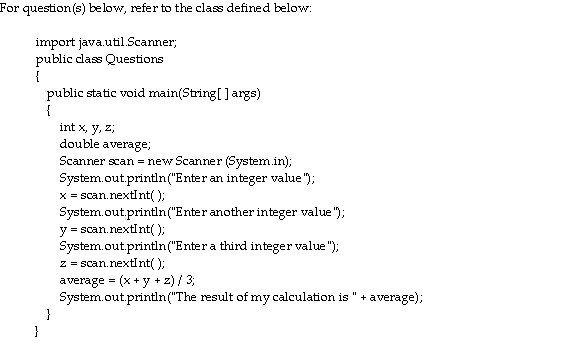19) What is output if x = 0, y = 1 and z = 1? 19) a b c d e
 A)  0.6666666666666666 B)  0.67 C)  0.0 D)  0 E)  0.6666666666666667

 20) What value will z have if we execute the following assignment statement? double z = 5 / 10; 20) a b c d e
 A)  z will equal 5.0 B)  z will equal 0.5 C)  z will equal 0.05 D)  z will equal 0.0 E)  none of the above, a run-time error arises because z is a double and 5 / 10 is an int

 21) What value will z have if we execute the following assignment statement? int z = 50 / 10.00; 21) a b c d e
 A)  10 B)  5.0 C)  50 D)  5 E)  none of the above, a compile or run-time error arises because z is an int and 50 / 10.00 is not

 22) A cast is required in which of the following situations? 22) a b c d e
 A)  storing numbers as a String B)  storing an int in a double C)  storing a double in a double D)  storing a double in an int E)  all of the above require casts

 23) If x is an int and y is a double, all of the following are legal except which assignment statement? 23) a b c d e
 A)  y = x; B)  x = y; C)  y = (double) x; D)  x = (int) y; E)  all of the above are legal

 24) What will be the value of a in the following assignment statement? Assume b = 5 and c = 10. int a = b * (-c + 2) / 2; 24) a b c d e
 A)  -30 B)  20 C)  30 D)  -20 E)  -6

 25) Of the following if statements, which one correctly executes three instructions if the condition is true? 25) a b c d e
 a.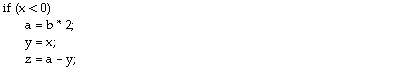b.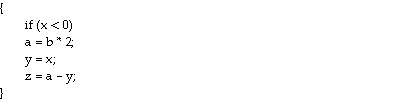c.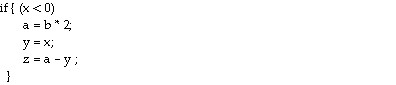d.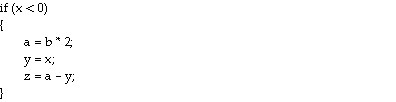e. E)  B, C and D are all correct, but not A

 26) Which of the sets of statements below will add 1 to x if x is positive and subtract 1 from x if x is negative but leave x alone if x is 0? 26) a b c d e
 a.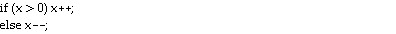b.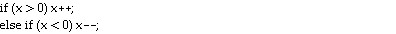c.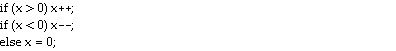d.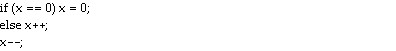e.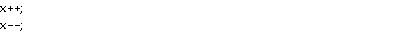Given the nested if-else structure below, answer the question(s) below.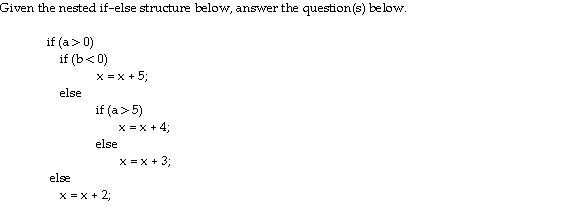27) If x is currently 0, a = 5 and b = 5, what will x become after the above statement is executed? 27) a b c d e
 A)  0 B)  2 C)  3 D)  4 E)  5

 28) If x is currently 0, a = 0 and b =  -5, what will x become after the above statement is executed? 28) a b c d e
 A)  0 B)  2 C)  3 D)  4 E)  5

 29) Consider the following code that will assign a letter grade of 'A', 'B', 'C', 'D', or 'F' depending on a student's test score. if(score >= 90) grade = 'A'; if(score >= 80) grade = 'B'; if(score >= 70) grade = 'C'; if(score >= 60) grade = 'D'; else grade = 'F'; 29) a b c d e
 A)  This code will work correctly only if score < 70 B)  This code will not work correctly under any circumstances C)  This code will work correctly in all cases D)  This code will work correctly only if score >= 60 E)  This code will work correctly only if score < 60

 30) Assume that count is 0, total is 20 and max is 1. The following statement will do which of the following? if (count != 0 && total / count > max) max = total / count; 30) a b c d e
 A)  The condition short circuits and the assignment statement is not executed B)  The condition short circuits so that there is no division by zero error when evaluating the condition, but the assignment statement causes a division by zero error C)  The condition does not short circuit causing a division by zero error D)  The condition will not compile because it uses improper syntax E)  The condition short circuits and the assignment statement is executed without problem

 31) Given two String variables, s1 and s2, to determine if they are the same length, which of the following conditions would you use? 31) a b c d e
 A)  (s1.equals(s2)) B)  (s1.length( ).equals(s2)) C)  (s1.length( ).equals(s2.length( )) D)  (s1.length( ) == s2.length( )) E)  length(s1) == length(s2)

 32) The following nested loop structure will execute the inner most statement (x++) how many times? for(int j = 0; j < 10; j++) for(int k = 10; k > 0; k--) x++; 32) a b c d e
 A)  100 B)  10 C)  20 D)  10,000 E)  20,000